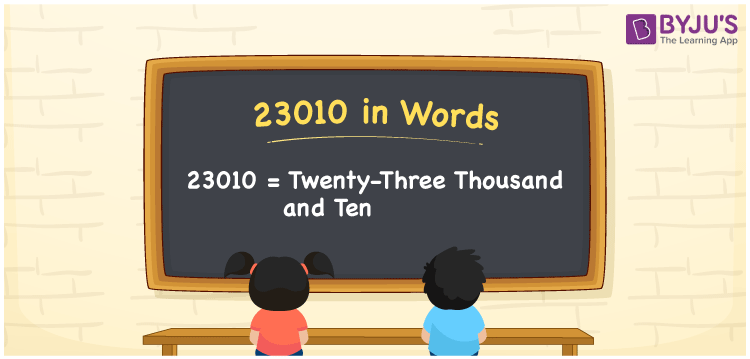# 23010 in words

23010 in words is written as Twenty Three Thousand and Ten. In 23010, the 2 has a place value of ten thousand, 3 is in the place value of thousand and 1 is in the place value of ten. The article on Place Value gives more information. The number 23010 is used in expressions that relate to money, distance, social media views, and many more. For example, “There are Twenty Three Thousand and Ten students across all branches of this school”.

 23010 in words Twenty Three Thousand and Ten Twenty Three Thousand and Ten in Numbers 23010

## 23010 in English Words## How to Write 23010 in Words?

We can convert 23010 to words using a place value chart. The number 23010 has 5 digits, so let’s make a chart that shows the place value up to 5 digits.

 Ten thousand Thousands Hundreds Tens Ones 2 3 0 1 0

Thus, we can write the expanded form as:

2 × Ten thousand + 3 × Thousand + 0 × Hundred + 1 × Ten + 0 × One

= 2 × 10000 + 3 × 1000 + 0 × 100 + 1 × 10 + 0 × 1

= 23010.

= Twenty Three Thousand and Ten.

23010 is the natural number that is succeeded by 23009 and preceded by 23011.

23010 in words – Twenty Three Thousand and Ten.

Is 23010 an odd number? – No.

Is 23010 an even number? – Yes.

Is 23010 a perfect square number? – No.

Is 23010 a perfect cube number? – No.

Is 23010 a prime number? – No.

Is 23010 a composite number? – Yes.

## Solved Example

1. Write the number 23010 in expanded form

Solution: 2 x 10000 + 3 x 1000 + 0 x 100 + 1 x 10 + 0 x 1

We can write 23010 = 20000 + 3000 + 000 + 10 + 0

= 2 x 10000 + 3 x 1000 + 0 x 100 + 1 x 10 + 0 x 1.

## Frequently Asked Questions on 23010 in words

Q1

### How to write the number 23010 in words?

23010 in words is written as Twenty Three Thousand and Ten.
Q2

### Is 23010 a prime number?

No. 23010 is not a prime number.
Q3

### Is 23010 divisible by 10?

Yes. 23010 is divisible by 10.